# RBSE Class 9 Science Chapter 11: Sound Important Textbook Questions and Solutions

There are different forms of energy like mechanical energy, electric energy, heat, light energy, nuclear energy etc. One more form of energy is sound energy. We hear sound from different sources in our day-to-day life. The sensation generated in our ears by the sound vibrations helps us to hear voices. RBSE Class 9 Science Chapter 11 solutions from BYJU’S help students, giving an excellent understanding of basic concepts related to Chapter 11 – Sound.

These textbook questions and solutions have been prepared by our subject experts to help the students in answering their queries relevant to the chapter. These RBSE Class 9 Science Chapter 11 solutions will help the students to cross-check their answers and learn from their mistakes. These solutions will also help students to relieve their anxiety so that they can get maximum scores in the exams.

Students can also get all subjects Class 9 solutions for Rajasthan Board here.

### RBSE Class 9 Science Chapter 11: Objective Textbook Questions and Solutions

Q1. The wave having compression and rarefaction is known as:

(A) Transverse wave

(B) Longitudinal wave

(C) Light wave

(D) Ultraviolet waves

Q2. Longitudinal waves can be generated in:

(A) solid and gas

(B) solid and liquid

(C) gas and liquid

(D) solid, liquid and gas

Q3.The vibration of the particles of the medium in longitudinal waves is:

(A) In the direction of the wave

(B) Vertical to the direction of the wave

(C)The particles do not vibrate

(D) at an angle of 60º to the direction of waves

Q4. The speed of sound is maximum in:

(A) air

(B) solid

(C) water

(D) Both water and solid

Q5. The time period of the hour-hand of a clock is:

(A) 1 hour

(B) 24 hours

(C) 12 hours

(D) None of the above

Q6. If the speed of a wave is 350 m/s and its wavelength is 50 cm then the frequency of the wave will be:

(A) 13500 Hz

(B) 700 Hz

(C) 400 Hz

(D) 300 Hz

Q7. The number of complete oscillations made in one second is known as:

(A) Amplitude

(B) Speed

(C) Time period

(D) Frequency

Q8. The time period of a vibrating body is 0.02 s. The frequency of vibrations will be:

(A) 100 Hz

(B) 20 Hz

(C) 50 Hz

(D) 1 Hz

Q9. The time period of the seconds-hand of a watch is :

(A) 1 minute

(B) 1 hour

(C) 12 hours

(D) 24 hours

Q10. The limit of audibility is:

(A) 200 Hz to 20,000 Hz

(B) 20 Hz to 20,000 Hz

(C) 2 Hz to 20 Hz

(D) more than 20,000 Hz

### RBSE Class 9 Science Chapter 11: Very Short Answer Type Questions and Solutions

Q11. Longitudinal waves can be generated in which type of medium?

Answer: Longitudinal waves can be generated in all three mediums, Solid, Liquid and Gas.

Q12. The sound waves produced in iron are of which type?

Answer: Sound waves produced in iron are Longitudinal waves.

Q13. The sound waves produced in air are of which type?

Answer: Sound waves produced in air are Longitudinal waves

Q14. If a wire tied between two pegs is stretched vertically to its length and is left, then which type of wave will be produced in the wire.

Answer: If a wire tied between two pegs is stretched vertically to its length and is left, then transverse type of wave will be produced in the wire.

Q15. What is the SI unit of wave length?

Answer: The SI unit of wavelength is Metre (m)

Q16. What is the SI unit of frequency?

Answer: The SI unit of frequency is Hertz (Hz)

Q17. Which type of waves will be generated if a freely hanging slinky is stretched and is left?

Answer: Longitudinal waves will be generated if a freely hanging slinky is stretched and is left.

Q18. What is the type of movement of the hands of a clock?

Answer: Periodic motion is the type of movement of the hands of a clock.

Q19. Define wavelength.

Answer: Wavelength is defined as the distance between the maximum displacement of amplitude from equilibrium. To put it in more simple terms, it is the space generated in a periodic wave—the distance over which the wave’s shape is repeated. It is usually measured or determined by taking the distance between corresponding points of the same phase or different spatial wave patterns. Wavelength is commonly expressed by the symbol λ which is actually a Greek letter known as lambd

Q20. Define frequency.

Answer: Frequency is defined as the number of oscillations of a wave per unit time being measured in hertz(Hz). The frequency is directly proportional to the pitch. Humans can hear sounds with frequencies ranging between 20 – 20000 Hz. Sounds with frequencies over the human ears range are known as ultrasound and sounds with frequencies less than the audible range are known as infra sound.

Answer: Radar is to send and receive valuable information in the form of waves. Radar is helpful in detecting incoming signals during a war and also used by geologists for earthquake detection. Archeologists use this technology for detection of buried artifacts. It is also used to understand the environment and climatic changes.

### RBSE Class 9 Science Chapter 11: Short Answer Type Questions and Solutions

Q22. What is essential for production of sound?

Answer: When something vibrates, the particles of the medium transfer their energy to the nearby particles of the medium by vibrating. As a result, the neighbouring particles start vibrating and similarly pass on their energy to their neighbouring particles. Thus the sound (energy) reaches our ears passing in the form of vibrations from particle to particle. In the ear these vibrations or disturbances generate stimuli because of which we are able to hear the

sound.

Q23. What do you understand by motion of a wave?

Answer: Wave motion is the transfer of energy and momentum from one point of the medium to another point of the medium without actual transport of matter between two points. Wave motion is classified into three different ways, which are,

• The medium of propagation
• The dimensions in which a wave propagates energy
• The energy transfer

Q24. What are longitudinal waves?

Answer: The particles of the medium vibrating about their equilibrium position in a direction parallel to the direction of propagation of the wave are called longitudinal waves. Longitudinal waves require a medium with only elasticity of volume (or Bulk modulus) for its propagation. In this type of wave motion, the waves travel through a medium in the form of compression and rarefaction.

Q25. What is required for the propagation of waves?

Answer: The substance through which the vibrations of objects pass is known as the medium. Sound is a mechanical wave and a medium like- air, water, iron etc. is required for its propagation. It cannot move in vacuum. Waves can be of two types, depending upon the direction of vibration and propagation: Transverse waves and Longitudinal waves.

Q26. What is transferred during wave propagation from one point to another? Energy or some physical mass?

Answer: Energy is transferred during wave propagation from one point to another.

Q27. Give two examples of longitudinal waves.

Answer: Longitudinal waves : The waves in which the vibrations are in the direction of the propagation are known as longitudinal waves. For example: waves generated in a spring, sound waves, etc. Sound waves are longitudinal waves.

Q28. Are waves generated by a bullet fired from a gun or a stone hurled from a catapult?

Answer: Yes, the waves waves generated by a bullet fired from a gun or a stone hurled from a catapult will follow wave motion.

Q29. Write the method of ‘position location’ by a radar and the uses of radar.

Answer: Radio waves are transmitted from radar and the time taken by them to return, on being reflected from the distant object is measured. The velocity of radio waves is 1,86,999 miles per second. Therefore the distance of the object can be easily calculated on knowing the time taken. The exact location of the reflector, that is, the target object is known by the high direction sensing antenna on the Radar. The actual position of the object becomes known by knowing the distance and direction. The transmitter of the radar emits momentary but intense pulses of radio waves at regular intervals. The receiver receives the reflected waves from outer objects, if present, in the time interval between the two pulses. The exact timing of reception of reflected waves is known by the electric circuits and the distance is then known instantly by the indicators marked in proportion to time.

Radars have a wide range of usage in military operations. They are used in Naval, Ground as well as Air defense purposes. They are used for detection, tracking and surveillance purposes also. Weapon control and missile guidance often use various types of RADARs.

These are used in Law Enforcements

Law enforcement, especially highway police, has an extensive uses of Radars during a pursuit in order to measure the speed of a vehicle. Due to bad weather conditions, when satellites are unable to get a clear image of traffic and barricades, then Radars are used to get the desired results.

This technology is used in Space

Radars are used to track and detect satellites and spacecraft. They are also used for safe landing and docking of spacecraft. Radars in satellites are used for remote sensing.

Radar is used for Remote Sensing of Environment

Just like various types of waves are received by an antenna. This technology is also used to detect weather conditions of the atmosphere and are also used for tracking motions of planets, asteroids and other celestial bodies in the solar system.

It is used in Aircraft Navigation

Ground mapping radars and weather avoidance radars are used in aircraft to navigate it properly. This technology enables an aircraft to ensure the location of obstacles, which can be a threat to the flight plan.

Q30. What is the difference between the longitudinal and the transverse waves?

 Difference Between Longitudinal and Transverse Wave Longitudinal Transverse The movement of the medium is of the same direction of the wave The movement of the medium is perpendicular to the direction of wave It acts in one dimension It acts in two dimension The wave cannot be polarized or aligned The wave can be polarized or aligned This wave can be produced in any medium such as gas, liquid or solid This wave can be produced in solid and liquid surface Earthquake P wave is an example Earthquake S wave is an example It is made of rarefactions and compressions It is made of troughs and crests

### RBSE Class 9 Science Chapter 11: Essay Type Questions and Solutions

Q31. Define the following:

(i) Amplitude

(ii) Frequency

(ii) Time period

(iv) Wave-length

Answer i: Amplitude – The amplitude of a wave is the maximum distance moved by the particles of a medium on either side of the mean position.

Answer ii: Frequency – We can define frequency of a sinusoidal wave as the number of complete oscillations made by any element of the wave per unit time. For a sinusoidal wave represented by the equation:

y (0,t) = -a

The formula of the frequency with the SI unit is given as:

Formula –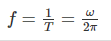SI Unit – Hertz

Answer iii: Time period – In sinusoidal wave motion, as shown above, the particles move about the mean equilibrium or mean position with the passage of time. The particles rise till they reach the highest point that is the crest and then continue to fall till they reach the lowest point that is the trough. The cycle repeats itself in a uniform pattern. The time period of oscillation of a wave is defined as the time taken by any element of the string to complete one such oscillation. For a sine wave represented by the equation: y (0, t) = -a sin(ωt)

The time period formula is given as: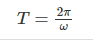Answer iv: Wavelength – The distance between consecutive crests of a wave, particularly the points of an electromagnetic wave is known as its wavelength.The wavelength and frequency are closely related. Higher the frequency, shorter is the wavelength. Since all the light waves move through a vacuum with same speed, the number of wave crests passing by a given point per second depends on the wavelength.

The Wavelength is denoted by λ. The Wavelength Formula of any wave is given by

#### λ = v/f

Where,

V= wave speed

f = frequency

The Wavelength is expressed in m, velocity is expressed in m/s, frequency is expressed in Hz.

Q32. Write the relation between:

(i) Time period and frequency

(ii) Frequency, wavelength and velocity

Answer i: Frequency represented as f, is the number of cycles of an oscillation that occur per second. It is measured in cycles per second or Hertz (hz).

Period of a wave represented as T, is the total time a wave takes to vibrate one full cycle.

Frequency and Period of a wave, both the terms, are inversely proportional to each other:

f = 1/T and T = 1/f.

Example 1: Given, Frequency is 2, calculate the time period:

As we know, The time period is the reciprocal of frequency,

So, Time Period = 1/Frequency = 1/2 = 0.5

Example – 2:

Given, Time Period is 4. Calculate the Frequency:

As we know, The frequence is the reciprocal of time period,

So, Frequence = 1/Time Period = 1/4 = 0.25

Answer ii: Frequency : “The number of vibrations or oscillations occurring in unit time is known as the frequency of the sound wave”. It is denoted by u and it’s SI unit is Hertz (Hz).

Wavelength: The distance between consecutive crests of a wave, particularly the points of an electromagnetic wave is known as its wavelength.The wavelength and frequency are closely related. Higher the frequency, shorter is the wavelength. Since all the light waves move through a vacuum with same speed, the number of wave crests passing by a given point per second depends on the wavelength.

Velocity: In a wave, the distance travelled in one second by a point (like compression or rarefaction) is known as the wave velocity.

The relation between wavelength, frequency and velocity is given by the formula,

c = v.Î»

Where, c is the velocity, v is the frequency and Î» is the wavelength.

(i) What is the distance travelled by the wave when the particle of medium completes one oscillation?

(ii) The sound waves in air are transverse or longitudinal?

(iii) Write the names of the wave/waves which can be generated in a long slinky.

(iv) Give two examples of each : the longitudinal waves and the transverse waves.

(v) Name the physical quantity whose unit is Hz.

Answer i: The distance travelled by the wave when the particle of medium completes one oscillation is equal to the wave length.

Answer ii: Sound waves in air are Longitudinal waves

Answer iii: The names of the wave/waves which can be generated in a long slinky are Longitudinal waves.

Answer iv: Examples of Transverse waves – light waves, energy transmitted in a stretched rope etc.

Examples of Longitudinal waves – waves generated in a spring, sound waves, etc.

Q34. Write the uses of ultrasound.

Answer: Ultrasound are high frequency waves. Ultrasound is extensively used in the field of medical science and in industries. Some of its uses are as follows:

(i) Ultrasound is used to find out the cracks and other defects in metallic blocks. Ultrasounds are passed through the metallic blocks and indicators are used to detect the transmitted waves. If there is any defect the ultrasound waves are reflected, which indicates defect in the block.

(ii) Ultrasounds are used to clean the objects which are not reachable. The objects to be cleaned are placed in the cleansing solution and ultrasound waves are passed. The particles of dust, grease and dirt separate and fall apart due to the high frequency.

(iii) Ultrasound waves are reflected from various parts of the heart and an image is formed. This is known as the electrocardiogram (ECG).

(iv) Ultrasound is also used in the ultrasound sonography. In it images of various organs like liver, gallbladder, uterus, kidney etc. are obtained using ultrasound waves. Ultrasonography is made use of to examine the embryo during pregnancy and to detect any irregularities. This technique is used to detect tumours. Ultrasound is also used to break the kidney stone into minute pieces so that they come out along with the urine.

Q35. Write the extended form of SONAR. How will you determine the depth of the sea by using reverberation range?

Answer: This is a device in which ultrasound is made use of to determine the distance, direction and speed of objects present in the water. The English synonym of the word SONAR is Sound Navigation and Ranging.

In sonar, there is a transmitter and a detector and it is put on the boat or ship. The ultrasound waves generated by the transmitter travels in water and after colliding with the object or sea floor are reflected. These reflected rays are detected by the detector which converts them into electric signals. The distance of the object from which the sound waves are reflected can be calculated by knowing the speed of sound (in water) and the time interval between transmission and detection of the ultrasound.

Suppose the time interval between transmission and detection of ultrasound signals is t and the speed of sound in marine water is v and if the object is at a depth d then the distance travelled from the ship to the object and back to the ship will be 2d. Then 2d = v × t.

Q36. Explain, with the help of a suitable diagram, the working of the human ear.

Answer: The outer ear is known as the ‘pinna’. It collects the sound from the surroundings and sends them to the auditory canal. On the end of the auditory canal there is a thin membrane called the ear drum. When the compression due to sound reaches the membrane the pressure on the outside of the membrane increases and pushes the ear drum inwards and at the time of rarefaction the membrane moves toward outside. Thus, vibrations are generated on the ear drum.

Three bones – the hammer, anvil and stirrup magnifies these vibrations manifold. The middle ear conducts these changes in pressure to the inner ears. The cochlea in the inner ear converts these pressure variations into electric signals and send them to the brain. The brain then interprets them in the form of sound.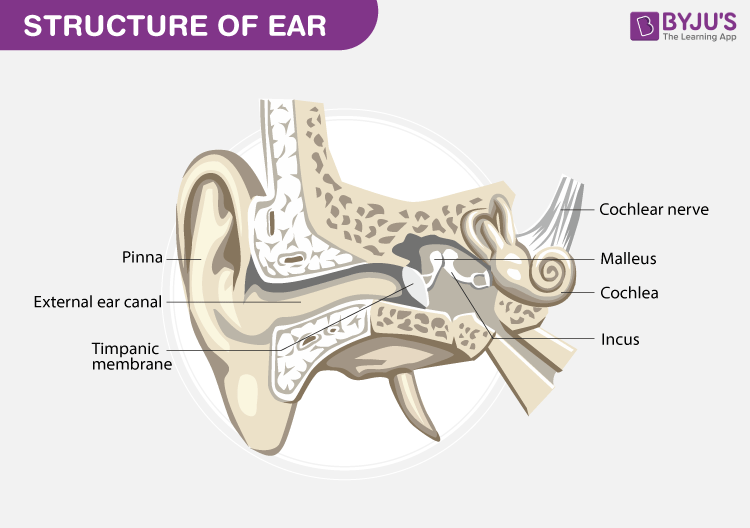Q37. Write the laws of reflection of sound. Describe an activity to verify them.

Answer: Reflections of sound – Just like the reflection of light, the reflection of sound is similar as it follows the laws of reflections, where the angle of reflection is equal to the angle of incidence and the reflected sound, the incident sound, and the normal sound belong in the same plane. Sound bounces off the surface of the medium which can be a solid or a liquid. In order to make the reflection of sound to occur, the surface can be of large size and can be either rough or polished.

Laws of Reflection of Sound

• The angle of reflection is always equal to the angle of incidence .
• The reflected sound, the incident sound and the normal sound belong in the same plane.

Activity: Two similar pipes are arranged near a wall, on a table, place a clock or some other source of sound at the open end of one of the pipes. Try to listen to this sound by placing your ear at the open end of the other pipe. Adjust the angle of the second pipe to hear the sound clearly. Now measure the angles of these pipes with the perpendicular drawn.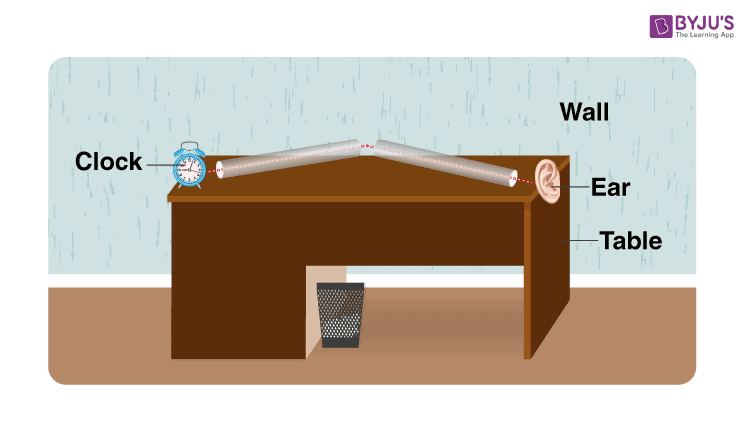We find that The sound is heard with utmost clarity when the angles made by the two with the perpendicular are equal i.e. ∠i = ∠r.

Q38. What is ultrasound? How can it be used to detect the defects in metallic blocks?

Answer: The sound whose frequency is more than 20,000 Hz (20KHz) are known as the ultrasound waves. They can be produced by the vibrations of the quartz crystal. Animals like bat, cat, dog etc. and some birds and insects use ultra sound waves for signaling. They are used to measure the depth of the oceans, in medical science and to remove fog at airports. In air the wavelength of these waves is less than 1.6 cm.

Ultrasound is used to find out the cracks and other defects in metallic blocks. Ultrasounds are passed through the metallic blocks and indicators are used to detect the transmitted waves. If there is any defect, the ultrasound waves are reflected which indicates defect in the block.

Q39. Elucidate the components of a radar and their functions.

Modulator: The essential impulse of high electric power provided to the Radio frequency Oscillator is obtained from the modulator.

Radio frequency Oscillator generates the high frequency power impulses from which the radar signals are formed. Antenna transmits these impulses to the sky and receives them back.

Indicator provides information gathered by the antenna to the radar operator.

Synchronisation and measurement of range is done by the modulator and the indicator.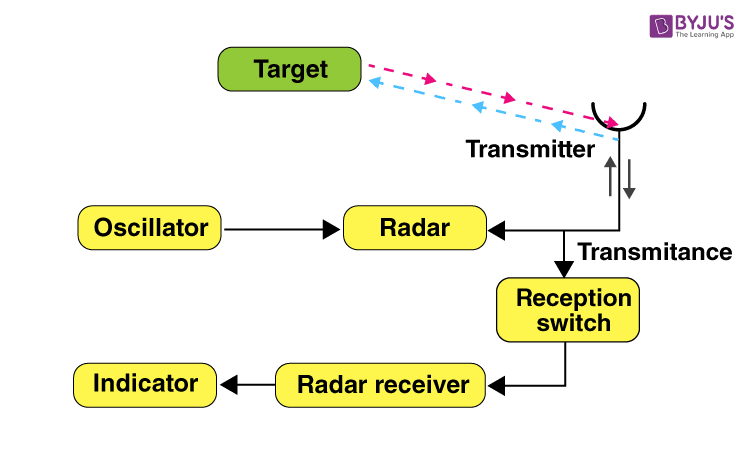• It is used in navigation of ships and aeroplanes
• In the GPS system
• While launching rockets and missiles.
• It helps in locating the enemy’s missiles, rockets and aircrafts.

Q40. Define the uses of Multiple Reflection of Sound.

Answer: Uses of Multiple Reflection of Sound:

• Loudspeakers, horns and musical instruments like shehnai are designed in a manner that the sound spreads in all directions. The sound waves produced by them are reflected many times and then are sent towards the listener.
• Stethoscope is an important instrument in medical research, which is used by doctors to listen to the sound of heart and lungs. In it the sound reaches the doctor’s ears by multiple reflections.
• The roof of the concert halls, seminar rooms and cinema halls is curved so that the sound reaches all parts of the hall after reflection.

Q41. Describe some facts regarding the characteristics of sound?

Answer: Some facts regarding the characteristics of sound are as under :

• Pitch is the property of sound because of which it is shrill or heavy. Pitch cannot be measured but can be experienced.
• Pitch depends on the frequency of the sound. The pitch of the high frequency sound is high. This sound is shrill to hear. On the other hand, the pitch of low frequency sound is low and is heavy sound.
• The pitch of buzzing mosquito is high while that of the roar of a lion is low.
• Loudness is the property of sound on the basis of which it is loud or soft to listen. The loudness of soft or quiet sound is less while that of the loud sound is more.
• The loudness of sound depends on the medium of sound propagation as well as the sensitivity of the ears. The same sound can be loud for one person while for the other it may be soft.
• Quality or timbre is characteristic of sound on the basis of which two sounds having the same pitch and loudness can be distinguished from each other.

Q42. What is Reverberation?

Answer: The sound produced in an auditorium or a large hall is repeatedly reflected by its walls causing persistence of the sound after its source has stopped. This repeated or multiple reflection, which results in cannot be heard. They are produced at the time of earthquakes, volcanic eruptions and in animals like whales, elephants etc.

### RBSE Class 9 Science Chapter 11: Numerical Questions and Solutions

Q43. An object is vibrating 6600 vibrations per minute. If the velocity of sound in air is 330 m/s then find:

(i) Time period

(ii) Frequency

(iii) Wave length

N or the Number of Vibrations per minute = 6600

Therefore, Number of Vibrations per second = 6600/60 = 110

V or S or Speed of sound in air per second = 330 m/s

We can calculate time by,

Time Period = 1 / N

= 1 / 110

= 0.0091.

Frequency = Time period

Wavelength = Velocity / Time period

Therefore, Wavelength = 330 / 1/110 = 3m

Q44. The time period of a body is 0.004 s. Find its frequency.

Time period = 0.004s

Time period = 1 / Frequency

Frequency = 1/time period

= 1/0.004

= 250 Hz

So, Frequency is 250 Hz

Q45. The distance between two adjacent crests of a wave is 30 cm and its frequency is 450 Hz. Then determine the velocity of the wave.

Wave length = 30cm

Frequency = 450 Hz

So, Wavelength = 30cm = 0.30m

Frequency = 450 Hz = 450s-1

Velocity = Wavelength × Frequency

= 0.30 × 450

= 135m/s

Therefore, the velocity of the wave is 135m/s

Q46. A wave of frequency 256 Hz is propagating at a speed of 330m/s. What will be the speed of a wave having frequency of 512 Hz, in the same medium?

Wave Frequency = 256Hz

Speed of the wave = 330Hz

By formula, we know that the

Speed = Frequency × Wavelength

Therefore, 330 = 256 × wavelength

Wavelength = 330/256

Now, since we know wavelength,

Speed = Frequency × Wavelength

Speed = 512 × 330/256 = 660m/s